# SSAT Upper Level Math : How to divide complex fractions

## Example Questions

← Previous 1

### Example Question #1 : How To Divide Complex Fractions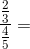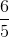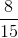Explanation:

Keep in mind that the main fraction line just means "divide."

So then,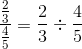Now, divide this like any other fraction.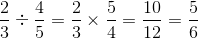### Example Question #2 : How To Divide Complex Fractions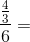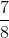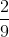Explanation:

Remember that the main line in the fraction means "divide."

Then,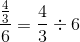Now, divide this like you would divide any other fraction.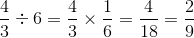### Example Question #3 : How To Divide Complex Fractions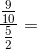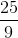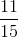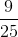Explanation:

Remember that the main line in the fraction means "divide."

Now,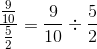Divide these two fractions.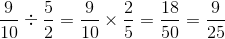### Example Question #4 : How To Divide Complex Fractions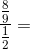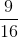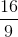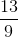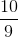Explanation:

The main fraction line means "divide."

Rewrite the complex fraction: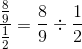Divide these fractions.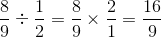### Example Question #5 : How To Divide Complex Fractions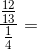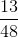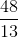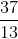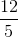Explanation:

The main fraction line means "divide."

Rewrite the complex fractions as thus: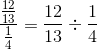Now, divide these two fractions.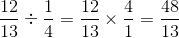### Example Question #6 : How To Divide Complex Fractions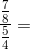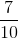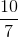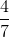Explanation:

The main fraction line means "divide." Rewrite the complex fraction as thus: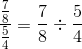Divide these two fractions.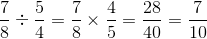### Example Question #7 : How To Divide Complex Fractions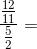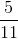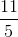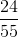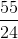Explanation:

The main line in a fraction means "divide." Rewrite the complex fraction as thus: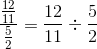Now, divide the fractions.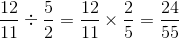### Example Question #8 : How To Divide Complex Fractions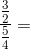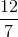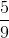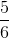Explanation:

The main line in a fraction means "divide." Rewrite the complex fraction as thus: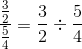Now, divide the fractions.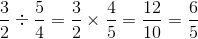### Example Question #9 : How To Divide Complex Fractions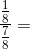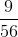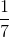Explanation:

The main line in a fraction means "divide." Rewrite the complex fraction as thus: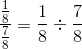Now, divide these fractions.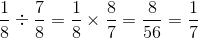### Example Question #10 : How To Divide Complex Fractions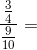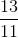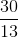Explanation:

The main line in a fraction means "divide." Rewrite the complex fraction as thus: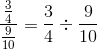Now, divide these fractions.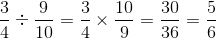← Previous 1